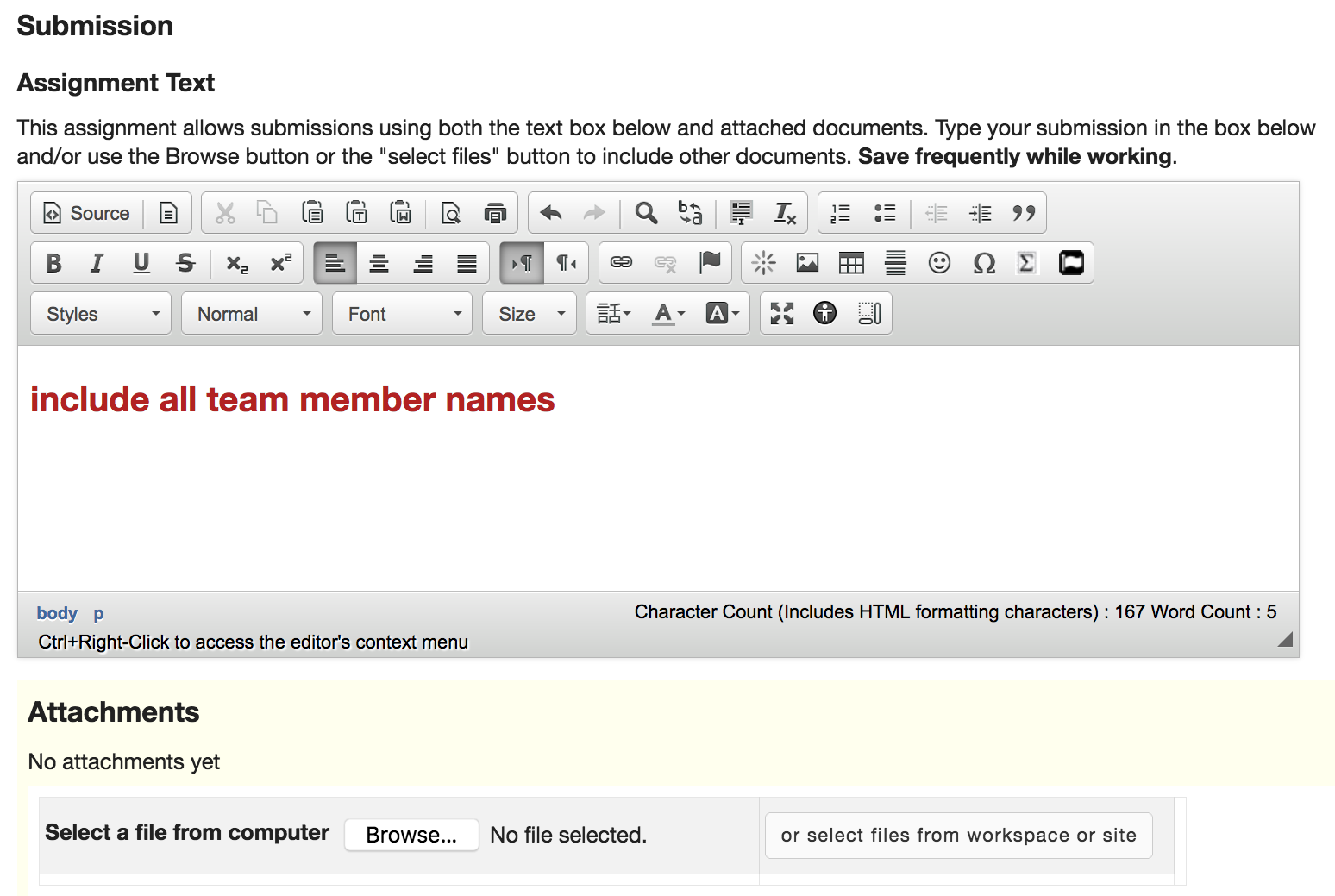## POTD 2: Get started with JS

Due 28-May-2021, 10:30am EST (no late submission, no extension)

Purpose: Introducing and Hands-on experience with JavaScript; get ready to work on homework assignment

For this activity, you may work alone or with another student in this class. Write JavaScript code to solve the following problems.

To run your program, you may:
• Use a web browser's console. For example, in Chrome — for Mac users, press command+option+J;  for Windows users, press Ctrl+shift+J.  Enter your code in the console, then press  Enter  key to run.
• Use JavaScript visualizer.  Enter your code in the editor, then press either  Visualize Execution  button or  Live Programming Mode  button. You may choose to run the entire code or run a step-by-step execution using  Previous  and  Next  buttons.
• Create an HTML file or use a template, embed your JavaScript in the HTML file (directly in the document or as an external file).

You may simply allow the script to be executed as the HTML page is loaded. Open the browser's console to see the result.

Alternatively, you may write HTML code to call the JavaScript function; you may need to modify your JavaScript such that the results are written as part of the HTML document.

### Problem #1: Simple Grid

Use  simplegrid.js, complete at least one of the functions: `isDiagonal`, `isEdge`, or `isMiddleRow`.

1. Imagine that the user specifies the width of a grid, as well as a tile. Complete the `isDiagonal` function to return either "diagonal" or "not diagonal" depending on whether or not the tile is along the diagonal of the square grid. For example, on a 7x7 grid, assuming the tile numbers (starting at the top-left corner) are 1, 2, 3, ..., 49

 1 2 3 4 5 6 7 8 15 22 23 24 25 26 27 28 29 30 36 43

all yellow tiles are on the diagonal.

You may use the following formulas in your program as needed:

```var row = Math.floor((tile-1)/width);   // Math.floor returns the largest integer less than or equal to a given number
var col = (tile-1) % width;             // %  returns the remainder of the division ```

2. Imagine that the user specifies the width and height of a grid, as well as a tile. Complete the `isEdge` function to return either "edge" or "not edge" depending on whether or not the tile is along the edge of grid. For example, on a 7x8 grid, assuming the tile numbers (starting at the top-left corner) are 1, 2, 3, ..., 56

 1 2 3 4 5 6 7 8 15 22 23 24 25 26 27 28 29 30 36 43 50

all yellow tiles are on the edge.

You may use the following formulas in your program as needed:

```var row = Math.floor((tile-1)/width);   // Math.floor returns the largest integer less than or equal to a given number
var col = (tile-1) % width;             // %  returns the remainder of the division ```

3. Imagine that the user specifies the width and height of a grid, as well as a tile. Complete the `isMiddleRow` function to return either "middle row" or "no" depending on whether or not the tile is along the middle row(s) of the grid. For example, on a 7x8 grid, assuming the tile numbers (starting at the top-left corner) are 1, 2, 3, ..., 56

 1 2 3 4 5 6 7 8 15 22 23 24 25 26 27 28 29 30 36 43 50

all yellow tiles are on the edge.

You may use the following formulas in your program as needed:

```var row = Math.floor((tile-1)/width);   // Math.floor returns the largest integer less than or equal to a given number
var col = (tile-1) % width;             // %  returns the remainder of the division ```

### Problem #2: Array and Loop

Use  array-loop.js, complete at least one of the functions: `sumList`, `findLast`, or `sum2D`.

1. Complete the `sumList` function that takes a non-empty list of integers, and then returns the sum of all elements in the list.
2. Complete the `findLast` function that takes a non-empty list of integers, and then returns the position of the last occurrence of the largest element.
3. Complete the `sum2D` function that takes a non-empty, square, two-dimentional array of integers, and then returns the sum of all the elements in the array.